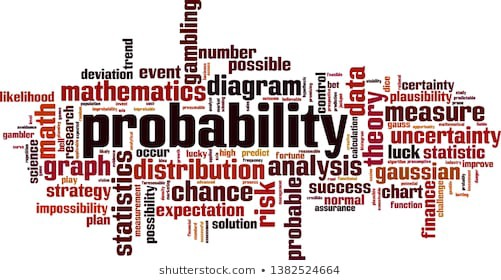If I am still blogging, chances are; I am still at the MBA, and I will be paying my next installment. Data Analysis is why I am asking this question again this week.

I will run down on the basics, just the way someone like me (who gets thrown off her feet by numbers) will understand

## Probability Again

Probability is a numerical measure of the likelihood that an event will occur

Its values are always assigned on a scale from 0 to 1.

A probability near zero indicates an event is quite unlikely to occur, while a probability near one indicates an event is almost certain to occur.

### Some aspects of probability

1. Experiments and the Sample Space
2. Assigning Probabilities to Experimental Outcomes
3. Events and their Probabilities
4. Some basic Relationships of Probabilities

An experiment is any process that generates well defined outcomes

The sample space for an experiment is the set of all experimental outcomes

An experimental outcome is also called a sample point.

## Assigning Probabilities

#### Basic Requirements for Assigning Probabilities

1. The probability assigned to each experimental outcome must be between 0 and 1 inclusively.
2. The sum of the probabilities for all experimental outcomes must be equal to 1.

Probabilities can be assigned based on the assumption of equally likely outcomes – Classical Method.

Assigning probabilities based on the experimentation or historical data – Relative Frequency Method

Judgement is also another way of assigning probabilities – Subjective Method

## Events and their Probabilities

#### Complement of an event

The complement of an event is defined to be the event consisting of all the sample points that are not in A.

#### Union of Two Events

The union of two events A and B is the event containing all sample points that are in A or B or both. The union of A and B is denoted by A u B.

##### Intersection of Two Events

Intersection of events A and B is the set of all sample points that are in both A and B. The intersection of events A and B is denoted by A n B.

The addition law provides a way to compute the probability of event A, or B, or both A and B occurring. The law is written as P(AuB) = P(A) + P(B) – P(AnB).

#### Mutually Exclusive Events

Two events are said to be mutually exclusive if the events have no sample points in common

Two events are mutually exclusive if, when one event occurs, the other cannot occur. If events A and B are mutually exclusive, P(AnB) = 0.

#### Conditional Probability

The probability of an event given that another event has occurred conditional probability. The conditional probability of A given B is denoted by P(A|B)

To be continued…## Class Learning Reflections (3)

in## Eyes in the Sky: Class Presentation – Exploring the Moral Lessons of Modern Warfare

in